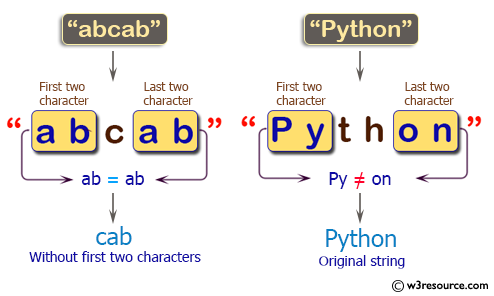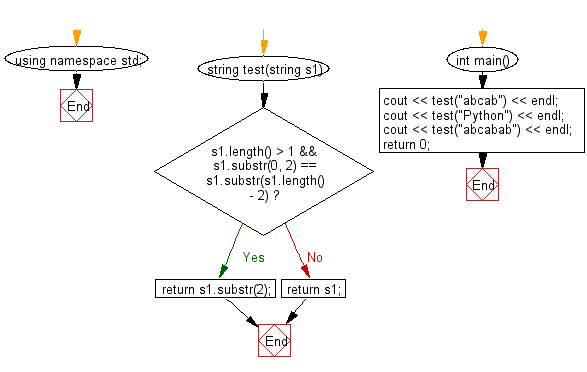﻿ C++ Exercises: Create a new string from a given string - w3resource# C++ Exercises: Create a new string from a given string

## C++ Basic Algorithm: Exercise-78 with Solution

Write a C++ program to create a new string from a given string. If the two characters of the given string from its beginning and end are same return the given string without the first two characters otherwise return the original string.

Sample Solution:

C++ Code :

``````#include <iostream>
using namespace std;

string test(string s1)
{
if (s1.length() > 1 && s1.substr(0, 2) == s1.substr(s1.length() - 2))
{
return s1.substr(2);
}
else
{
return s1;
}
}

int main()
{
cout << test("abcab") << endl;
cout << test("Python") << endl;
cout << test("abcabab") << endl;
return 0;
}
``````

Sample Output:

```cab
Python
cabab
```

Pictorial Presentation:Flowchart:C++ Code Editor:

Contribute your code and comments through Disqus.

What is the difficulty level of this exercise?

﻿